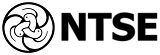National Talent Search Examination (NTSE) is a national-level scholarship program in India to identify and recognize students with high intellect and academic talent. It is one of the most prestigious exams in India. Close to 50,00,000 students (a largely self selective group of students) appear in this scholarship exam every year, and 1000 scholarships are awarded, out of which 775 are not reserved. Source : https://en.wikipedia.org/wiki/National_Talent_Search_Examination
Coordinate Geometry, Algebra, Mensuration - Perimeter, Area, Volume, etc., Data Handling, Number System, Geometry, Heron's Formula, Quadrilaterals, Logical Reasoning, Linear Equations in Two Variables, Rational Numbers, Real Numbers, Congruence of Triangles, Euclid's Geometry , Constructions , Area, Percentages, Quadratic Equations, Exponents and Powers, Linear Equations In One Variable, Triangles, Functions

Trigonometry, Arithmetic Progressions, Data Handling, Real Numbers, Logical Reasoning, Quadratic Equations, Linear Equations in Two Variables, Algebra, Mensuration - Perimeter, Area, Volume, etc., Geometry, Time, Coordinate Geometry, CIRCLE, Euclid's Geometry , Triangles, Constructions , Probability, Complex Numbers, Logarithm, Geometric Progression, Circles and Parabola, Slope, Functions, Permutation And Combination, Exponents and Powers, Graphs